# Math Games You’ll Love Using In The Classroom

Math games are a non-threatening and motivational way to provide additional practice to help consolidate math skills. I love these low tech versions!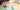Recently, I ran a spelling game challenge that you loved, so I thought you might want some math games to add to your teaching tools too. Math games are a non-threatening and motivational way to provide additional practice to help kids consolidate math skills. Yes, there are tons of online math games, but there’s something to be said for low tech versions … games that often have kids playing in pairs or small groups. (Want even more games and activities for your classroom? Check out the Top Notch Teaching Members Club.

## 10 classroom math games to love

I’ve pulled together 10 games that cover shape, adding on, estimating, mixed operations, place value, and more. These games are easy to set up and many require only things you probably use in your math lessons anyway—paper, pencil, (or white board and marker) and calculator. A deck of cards and some dice might be useful too. Any additional materials are noted in the instructions for each game.

### 1. 2D shape dominoes

The classic game of dominos is adapted as a math game. Use special dominos with 2D shapes or shape words.

Note: Print the dominoes onto thick cardstock. You don’t want the image visible through the paper when the dominos are turned upside down.

• Mix the dominoes and spread face down on the table.
• Each player chooses 7 dominoes and keeps them in front of them but hidden from other players.
• The youngest player begins by selecting one of their dominoes and placing it face up in the middle of the playing surface.
• The player to the left takes a turn and must place a matching domino next to the first domino. For example, if the first player started the game with the domino that has the word triangle and picture of an octagon, then the next player must play a domino that has a picture (or word) of a triangle or an octagon. They play their domino by placing matching parts side by side.
• If the player doesn’t have a matching domino, they pick up one domino from the draw pile. A turn is over once a player plays or draws.
• Play continues until one player uses all their dominoes and that player is the winner.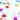### 2. Counting on card game

This two-player game helps students practice the mental math strategy of counting on.

Supplies: A deck of cards with the picture cards (King, Queen, Jack, Joker) removed.

Notes: Aces act as 1s in this game.

• Divide the cards into two piles, one with the cards: ace – 4 and the other pile with the cards 5 – 10.
• Shuffle each pile and place them face down on the playing surface.
• Players take turns turning over the top two cards and adding the two numbers using the counting on strategy: count on from the larger number, and count on the smaller number. For example, if the two cards turned over were 7 and 5. They would start with 7 and count on 5: 8, 9, 10, 11, 12.
• If a player has the correct answer, they get to keep both cards.
• If the answer is incorrect, the other player tries counting on. If they are correct, they get to keep both the cards.
• Play ends when one of the piles runs out of cards and the player with the most cards wins.

### 3. Card turnover multiplication or addition game

This 2-player game works for addition or multiplication.

Supplies: A  deck of cards with the picture cards (King, Queen, Jack, Joker) removed.

Notes: Aces act as 1s in this game.

• Shuffle the cards and divide them into two equal piles. Place the piles face down on the playing surface in front of each player.
• Each player flips a card from the top of their pile.
• Players multiply (or add) the two numbers, and the first player to call out the correct answer is the winner. That player gets to keep both cards. For example, if the cards turned up are a 6 and a 3, then the problem becomes: 6 x 3. Whichever player calls out 18 first, keeps the two cards. [If you are practicing addition, have students determine the sum of the two numbers instead. So the problem becomes 6 + 3 and the player to say 9 first wins the cards.]
• If both players say the correct answer at the same time, they each keep one card each.
• If neither player has the correct answer, then both cards are placed back at the bottom of the piles.
• The winner is the player with the most cards at the end.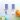### 4. I spy with my little eye

This 2-player card game provides practice for addition, but you can adapt it to practice subtraction, multiplication, or division.

Supplies: A deck of cards with the picture cards (King, Queen, Jack, Joker) removed.

Notes: Aces act as 1s in this game.

• The cards are dealt face up in either a 10 x 4 or 8 x 5 array.
• The first player says, “I spy with my little eye two cards that add to make ______.”
• The second player then looks for 2 cards that add to make the number. The two cards to be added need to be next to each other either horizontally or vertically (you can also choose to allow cards to be adjacent diagonally). The player then picks the cards up and adds them to their pile. They do this with any other pairs that add to make the number as well.
• If the second player misses any pairs that add to the number, then player 1 may claim them.
• The players alternate taking turns and continue until all the cards are gone.
• The winner is the player with the most cards at the end of the game.
• As large gaps appear in the array, move the cards closer together to fill those gaps.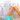### 5. Number sentence race

In this dice game for partners or small groups, students practice writing number sentences using addition, subtraction, multiplication, and division.

Supplies: Pencil and paper for each student, two dice per group, stopwatch or 4-minute timer.

• One student rolls the dice and adds the numbers together. (e.g. 6 + 6 = 12).
• Start the 4-minute timer.
• Students write as many number sentences for the number as they can.
For example:
5 + 7
20 – 8
4 x 3
24 / 2
12 + 0 etc.
• When time is up, each student scores their number sentences:
– Addition sentences are worth 1 point.
– Multiplication sentences are worth 2 points.
– Subtraction sentences are worth 3 points.
– Division sentences are worth 4 points.
• The student with the most points when the timer ends is the winner.

### 6. Place value dice

In this 2-person game, students create and compare numbers to practice place value.

Supplies: One die between two students and paper and pencils.

• Have students draw a place value table. The size of the table depends on the level of your students. In this example, we’ll use ones, tens, hundreds and thousands.
• One student rolls the die.
• Each student decides which place value spot they want to put the number in: ones, tens, hundreds or thousands slot.
• The next student rolls the die and again both students place the numbers in the place value table.
• When all the columns have been filled, students compare numbers. The higher number wins. Challenge them to see if they could create an even higher number by rearranging the order of the numbers they used.
• Variation: You can use cards instead of dice. Just remove face cards and 10s.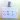### 7. Just 100

In this 2-person game, students practice addition and subtraction trying to get to exactly 100.

Supplies: One die between two students and paper and pencils.

• Students take turns rolling a die. They can add or subtract the number until they get to 100.
• Options: They can do the math mentally or you can have them show each equation.
• Variation: You can try with two dice as well. Students can decide to add both, subtract both or add one and subtract the other.

### 8. Knock out

In this game, students add, subtract, multiply, and divide to cross out all the numbers on their grid.

Supplies: A number grid 1–10, two dice and a pencil per student.

• One student rolls both dice.
• They can use any operation (addition, subtraction, multiplication, or division) to get one of the numbers. For example, if they roll 6 and 2, they could knock out 8, 4, 3.
6 + 2 = 8
6 – 2 = 4
6 / 2 = 3
(6 * 2 = 12, which is not on the grid)
• Variation: Younger students can play using only addition and subtraction.
• Go further: Ask students to predict which numbers will be the hardest to get. Which do they think will be easiest? Then ask how many ways they can find to knock out a particular number.

### 9. Aim for 9

In this game for pairs or small groups, students practice creating number sentences that combine operations to equal a single digit number. The higher the number, the higher the score. Thus aim for 9.

Supplies: 4 dice for each group, paper and pencil for each student.

• One player rolls all 4 dice. These are the 4 numbers students have to work with.
• Each player writes down a number sentence where the answer is a single digit. Students can only use each number once.
Example: the numbers rolled are: 1, 4, 3, 6.
Some possible number sentences:
6 + 4 + 1 – 3 = 8
4 x 1 + 6 – 3 = 7
41 – 36 = 5
[Note that students can use the numbers rolled to create double digit numbers.]
• After 5 rounds, the student with the most points is the winner.

### 10. Are you in the right range?

This 2-player game provides practice of the mental math skill – estimating.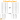Supplies: A calculator, 2 x 10-sided dice, and a copy of a playing board, which includes numbers to suit the level of your students (see sample below).

Note: Because this is an estimation game, students do not need paper and pencil to work out the sum.

• Player 1 rolls one die and finds the corresponding numbers on the playing board. They then roll the second die and find the number in the second box on the playing board. For example, if the player rolled 7 and 5, then they find 564 + 466.
• Player 1 estimates the sum of the two numbers and selects the range that the estimate falls in.
• The other player uses the calculator to work out the exact answer. If this answer falls within the range selected then player 1 scores a point.
• Player 2 takes a turn estimating and player 1 finds the exact answer using the calculator.
• Players continue swapping turns until one player gets 5 points and is declared the winner.

## Want more math games?

Not to mention spelling games, literacy activities, science experiments and PE lesson plans …? The Top Notch Teaching Members Club provides a wealth of on-demand teaching resources, advice, materials and learning programs to save you time.

Each month, new products are added, including spelling and math games not available anywhere else. You’ll also find time management tips, advice on avoiding teacher stress and burnout, and special perks and offers from my partners. Save time by having the teaching resources you need for a wide range of subjects at your fingertips.

#### Get even more details and join here >> https://topnotchteaching.com/members/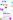## Why I Love Task Cards For Teaching Multiplication

Teaching multiplication goes beyond having children memorize multiplication tables. To build math fluency, try these best ways to teach multiplication.

## 6 Great Ideas For Teaching Place Value

Teaching place value is important for students. Place value helps us to read whole numbers into the millions and beyond. Try these place value activities.